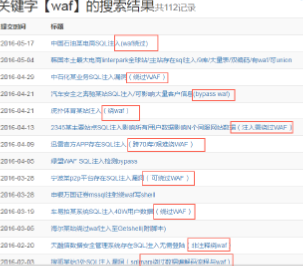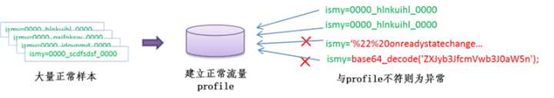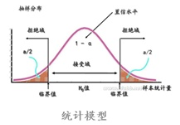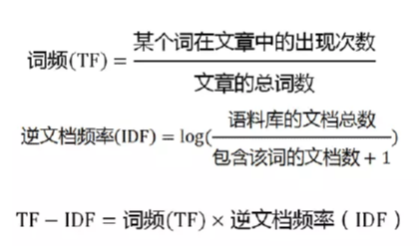## 一、背景## 二、技术思路

### 1.基于数据统计的模型### 2.基于文本分析的机器学习模型1）基于隐马尔科夫模型(HMM)的参数值异常检测。

2）基于TF-IDF的异常检测1. 先取得URL里的关键词列表
2. 用 scikit learn的 TfidfVectorizer函数把每个URL里的关键词做TF-IDF得到向量化的特征
3. 用 LogisticRegression 模型训练并进行评价。

3）基于词法分析和语法分析

4）基于色谱样熵分析(Chromatography-Like Entropy Analysis)

### 3.基于单分类模型### 4.基于聚类模型## 三、建模与测试

```import numpy as np

import urllib

# 数据预处理

directory = "C:\\fwaf"

filepath = directory + "\\" + filename

data = list(set(data))

queries = set()

for d in data:

d = d.strip()

try:

d = str(urllib.unquote(d).decode('utf8'))   #converting url encoded data to simple string

except:

print 'decode ' + d + ' error'

return list(queries)

tempvalidQueries = getQueryFromFile('goodqueries.txt')

ygood = np.zeros(len(tempvalidQueries))

queries = tempAllQueries

#tokenizer function, this will make 3 grams of each query

#构造3-gram特征

def getNGrams(query):

tempQuery = str(query)

ngrams = []

for i in range(0,len(tempQuery)-3):

ngrams.append(tempQuery[i:i+3])

return ngrams

from sklearn.feature_extraction.text import TfidfVectorizer

from sklearn.cross_validation import train_test_split

#converting data to vectors

#TF-IDF

vectorizer = TfidfVectorizer(tokenizer=getNGrams)

X = vectorizer.fit_transform(queries)```

```#splitting data

X_train, X_test, y_train, y_test = train_test_split(X, y, test_size=0.2, random_state=42)

from sklearn.linear_model import LogisticRegression

lgs = LogisticRegression()

lgs.fit(X_train, y_train) #training our model

print(lgs.score(X_test, y_test))  #checking the accuracy```

```X_predict = [

'AND 1=1',

'ORDER BY 1-- ',

'and (select substring(@@version,1,1))=\'X\'',

'www.baidu.com',

'<?php @eval(\$_POST[\'c\']);?>'

]

X_vecpredict = vectorizer.transform(X_predict)

y_Predict = lgs.predict(X_vecpredict)

#printing predicted values

0:'Good'}

for i in range(len(X_predict)):

print mapvalues[y_Predict[i]]+':'+X_predict[i]```

```Bad :ORDER BY 1--

Good:www.baidu.com

## 四、总结与展望

web流量异常检测只是web入侵检测中的一环，用于从海量日志中捞出少量的“可疑”行为，但是这个“少量”还是存在大量误报，只能用于检测，还远远不能用于WAF直接拦截。一个完备的web入侵检测系统，还需要在此基础上进行入侵行为识别，以及降低误报等环节。

1.C&C随机域名检测

2.恶意URL检测（可以参考新加坡大学的一个团队综述https://arxiv.org/abs/1701.07179

3.UGC违规图片与评论 机器学习在安全领域的广泛应用还有很长的路要走。

https://zhuanlan.zhihu.com/p/25139556

https://github.com/faizann24/Fwaf-Machine-Learning-driven-Web-Application-Firewall